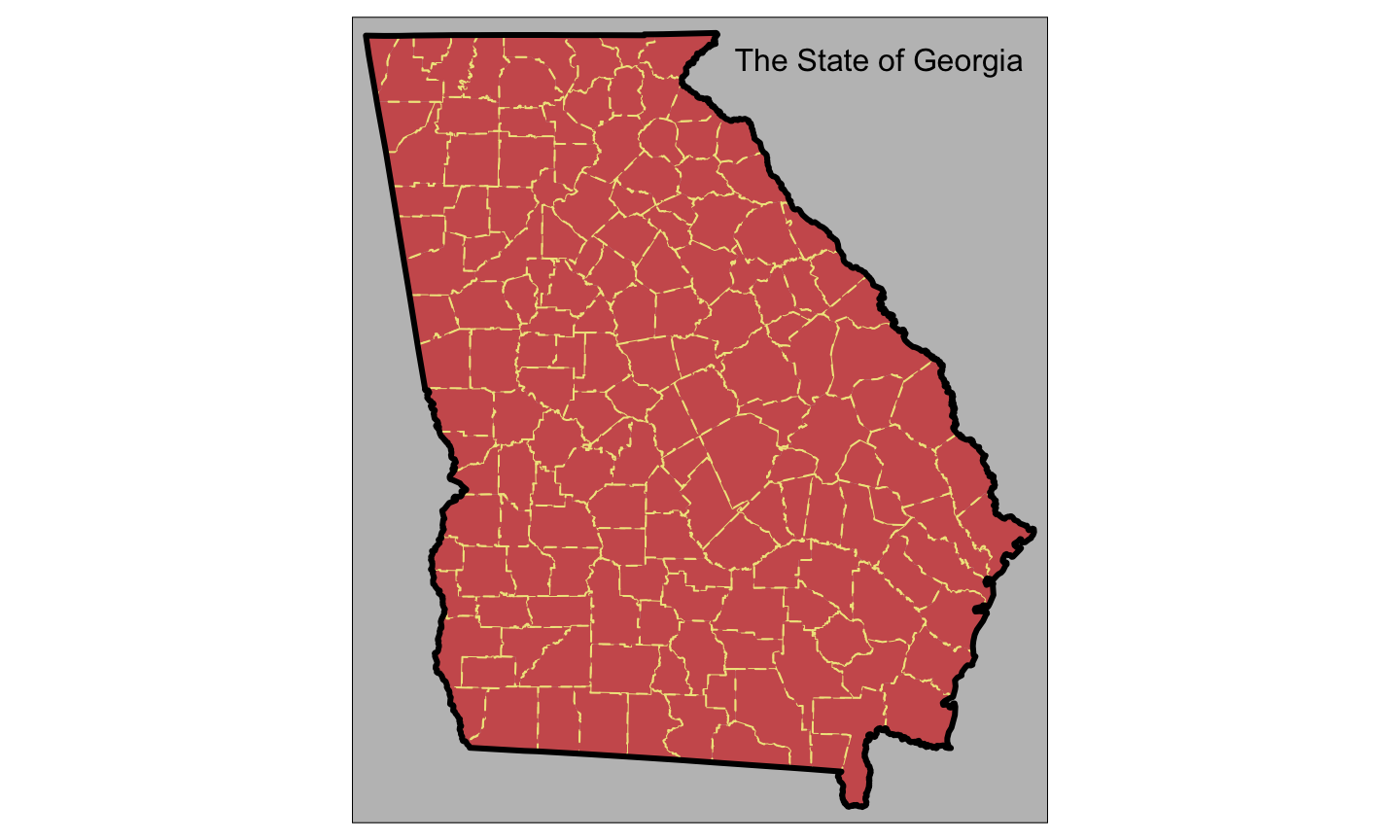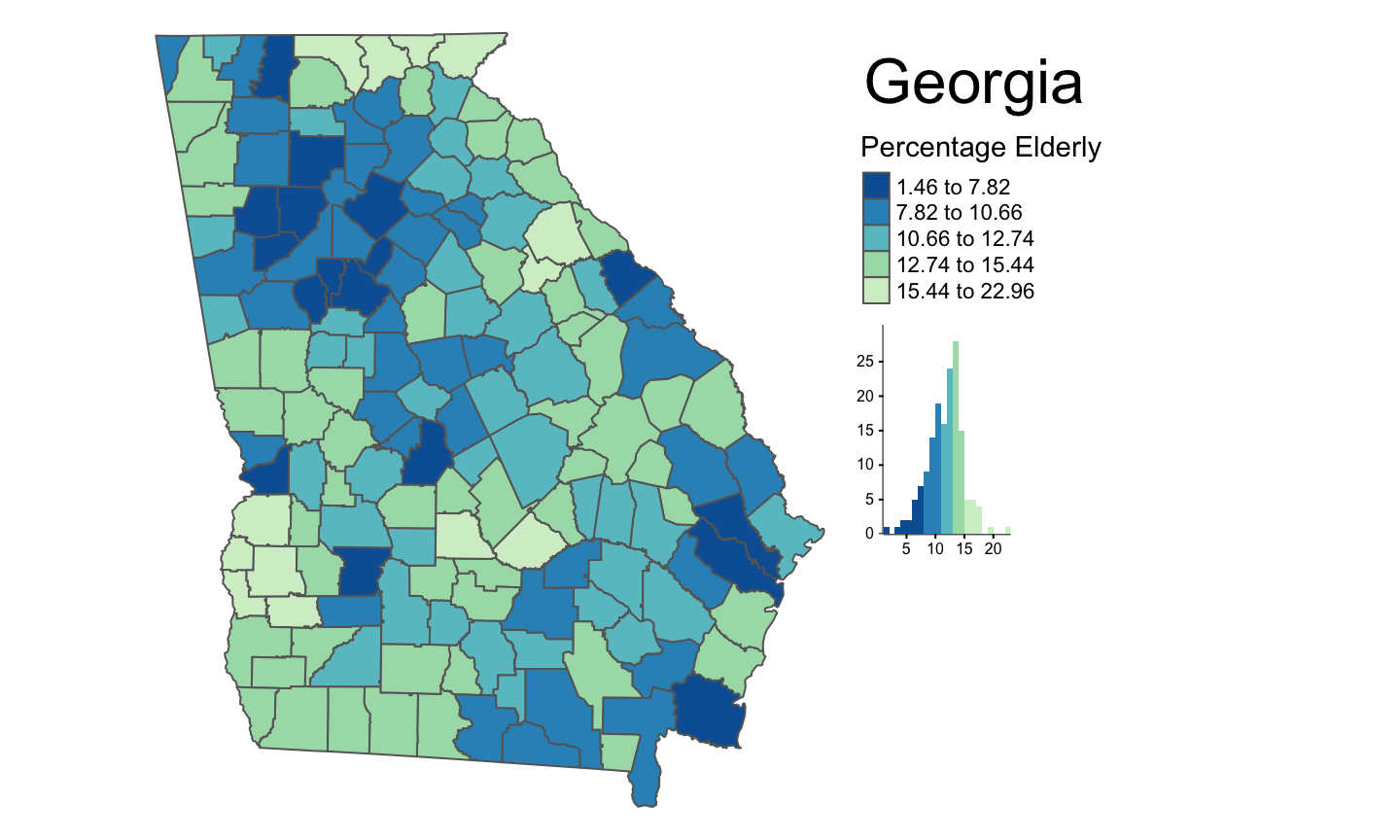July 2018

## 1. Introduction

• This is going to be a very short introduction talk
• you have a lot of ground to cover in the worksheet
• Spatial data formats in R (`sp` and `sf`)
• Reading and writing spatial data in and out of R
• Mapping with `tmap`
• Cluster analysis (hotspots and coldspots)
• Saving maps as PDFs, PNGs, TIFFs etc

## 1. Introduction

• you have already made developed some visualisations of data attributes held in spatial data
• the `tb` object in Session 3
```df <- as.tibble(data.frame(georgia))
tb <- as.tibble(df)```
• the `georgia` data is a `SpatialPolygonsDataFrame`
`class(georgia)`
```##  "SpatialPolygonsDataFrame"
## attr(,"package")
##  "sp"```

## 1. Introduction

• In this session you will explore two spatial data formats
• the old: `sp`
• the new: `sf`
• they both hold the geometry of the geographic features and the attribute values
• a bit like shapefiles
• however the plan is for `sf` to supersede `sp`
• hence the `sf` revolution
• we work mainly with `sf` but you will see that for some analyses we still have to work with `sp` at the moment.

## 2. Reading and writing spatial data

• for both `sf` and `sp`
• to read / write `georgia.shp` with `sf`
```setwd("/Users/geoaco")
st_write(g2, "georgia.shp", delete_layer = T)```
• to read / write `georgia.shp` with `sp`
```new.georgia <- readOGR("georgia.shp")
writeOGR(obj=new.georgia, dsn=".", layer="georgia",
driver="ESRI Shapefile") ```

## 3. Mapping with `tmap`

• maps built up as a series of layers
• exercise control
• like with `ggplot`
```tm_shape(georgia_sf) +
tm_fill("indianred") +
tm_borders(lty = "dashed", col = "khaki") +
tm_style_natural(bg.color = "grey75") +
tm_shape(g) +
tm_borders(lwd = 3) +
tm_layout(title = "The State of Georgia",
title.size = 1, title.position = c(0.55, "top"))```

## 3. Mapping with `tmap`

• maps built up as a series of layers
• exercise control
• like with `ggplot`## 3. Mapping with `tmap`

• you will also construct some choropleth maps of variables
• for polygons / areas## 3. Mapping with `tmap`

• you will also construct some choropleth maps of variables
• for points